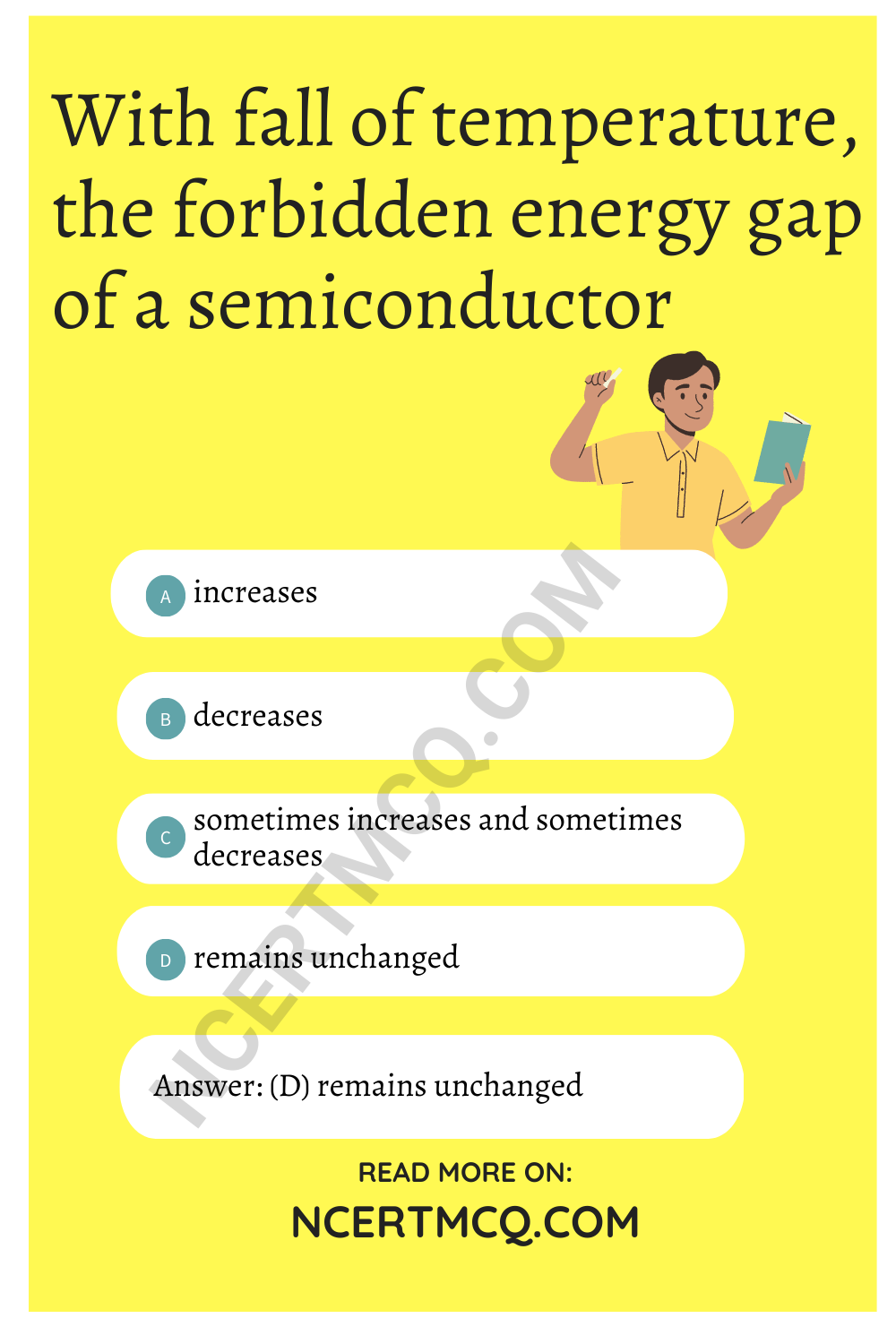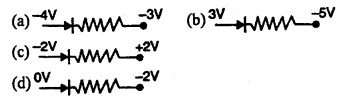Check the below Online Education NCERT MCQ Questions for Class 12 Physics Chapter 14 Semiconductor Electronics: Materials, Devices and Simple Circuits with Answers Pdf free download. MCQ Questions for Class 12 Physics with Answers were prepared based on the latest exam pattern. We have provided Semiconductor Electronics: Materials, Devices and Simple Circuits Class 12 Physics MCQs Questions with Answers to help students understand the concept very well.

## Semiconductor Electronics: Materials, Devices and Simple Circuits Class 12 MCQs Questions with Answers

MCQ On Semiconductor Class 12 Chapter 14 Question 1.
Bonds in a semiconductor :
(a) trivalent
(b) covalent
(c) bivalent
(d) monovalent

Semiconductor Class 12 MCQ Chapter 14 Question 2.
Number of electrons in the valence shell of a semiconductor is:
(a) 1
(b) 2
(c) 3
(d) 4

Class 12 Semiconductor MCQ Chapter 14 Question 3.
Semiconductors of both p-type and n-type are produced by:
(a) ionic solids
(b) covalent solids
(c) metallic solids
(d) molecular solids

Semiconductor MCQ Class 12 Chapter 14 Question 4.
With fall of temperature, the forbidden energy gap of a semiconductor
(a) increases
(b) decreases
(c) sometimes increases and sometimes decreases
(d) remains unchangedIt can solve systems of linear equations or systems involving nonlinear function calculator.

Semiconductor Class 12 MCQ Pdf Chapter 14 Question 5.
In a p-type semiconductor, current conduction is by:
(a) atoms
(b) holes
(c) electrons
(d) protons

MCQ Of Semiconductor Class 12 Chapter 14 Question 6.
The relation between number of free electrons (n) in a semiconductor and temperature (T) is given by:
(a) n ∝ T
(b) n ∝ T²
(c) n ∝ T1/2
(d) n ∝ T3/2

Semiconductor MCQs Class 12 Chapter 14 Question 7.
In reverse biasing:
(a) large amount of current flows
(b) no current flows
(c) potential barrier across junction increases
(d) depletion layer resistance increases

Answer: (c) potential barrier across junction increases

Chapter 14 Physics Class 12 MCQs Chapter 14 Question 8.
Main function of a transistor is to :
(a) rectify
(b) simplify
(c) amplify
(d) all the above

Semiconductor Questions And Answers Pdf Class 12 Chapter 14 Question 9.
To obtain p-type silicon semiconductor, we need to dope pure silicon with:
(a) aluminium
(b) phosphorus
(c) oxygen
(d)germanium

MCQ Semiconductor Physics Chapter 14 Question 10.
On applying reverse bias to a junction diode, it:
(a) lowers the potential barrier
(b) raise the potential barrier
(c) increases the majority carrier current
(d) increases the minority carrier current

Answer: (b) raise the potential barrier

Semiconductor MCQ Chapter 14 Question 11.
For germanium crystal, the forbidden energy gap in joules
(a) 1.216 × 10-19
(b) 1.76 × 10-19
(c) 1.6 × 10-19
(d) zero

Semiconductors Class 12 MCQ Chapter 14 Question 12.
To obtain electrons as majority charge carriers in a semiconductors the impurity mixed is:
(a) monovalent
(b) divalent
(c) trivalent
(d) pentavalent

Semiconductor Physics MCQ Chapter 14 Question 13.
In the middle of the depletion layer of a reverse biased p-n junction, the:
(a) electric field is zero
(b) potential is maximum
(c) electric field is maximum
(d) potential zero.

In a common base amplifier the phase difference between the input signal voltage and output voltage is :
(a) π/2
(b) 0
(c) π/4
(d) π

MCQ On Semiconductor Chapter 14 Question 15.
Energy bands in solids are a consequence of:
(a) Ohm’s Law
(b) Pauli’s exclusion principle
(c) Bohr’s theory
(d) Heisenberg’s uncertainty principle

Semiconductor MCQ Questions Chapter 14 Question 16.
In semi conductor which are responsible for conduction:
(a) only electron
(b) electron and hole both
(c) only hole
(d) None of these

Answer: (b) electron and hole both

Semiconductor Devices MCQ Chapter 14 Question 17.
In binary system III represents:
(a) 1
(b) 3
(c) 7
(d) 100

Semiconductors MCQ Chapter 14 Question 18.
On heating, resistance of semiconductors:
(a) decreases
(b) increases
(c) remains same
(d) first increases then decreases

MCQ Questions On Semiconductors Chapter 14 Question 19.
p-n junction diode can be used as:
(a) amplifier
(b) oscillator
(c) detector
(d) modulator

Question 20.
In intrinsic semiconductor at room temperature, the number of electrons and holes are:
(a) equal
(b) unequal
(c) infinite
(d) zero

Question 21.
A forward biased diode is:Question 22.
In full wave rectifier, input a.c. current has a frequency v. The output frequency of current is :
(a) V/2
(b) V
(c) 2V
(d) None

Question 23.
Winch of the following gate is not an universal gate?
(a) OR
(b) NOT
(c) AND
(d) NAND

Question 24.
Zener diode is used for:
(a) producing oscillations in a oscillator
(b) amplification
(c) stabilisation
(d) rectification

Question 25.
In semi conductor, at room temperature :
(a) the valence bond is partially empty and the conduction band is partially filled
(b) the valence band is completely filled and the conduction band is partially filled
(c) the valence band is completely filled
(d) the conduction band is completely empty

Answer: (a) the valence bond is partially empty and the conduction band is partially filled

Question 26.
Crystal diode is:
(a) amplifying device
(b) fluctuating device
(c) non-linear device
(d) linear device

Question 27.
The part of a transistor which is heavily doped to produce a large number of majority carriers is :
(a) base
(b) emitter
(c) collector
(d) None of these

Question 28.
A p-type semiconductor is:
(a) negatively charged
(b) positively charged
(c) uncharged
(d) None of these

Question 29.
The material most commonly used to manufacture electronic solid state devices is :
(a) copper
(b) silicon
(c) germanium
(d) aluminiumQuestion 30.
What is the number of possible crystal systems?
(a) 5
(b) 7
(c) 14
(d) 16